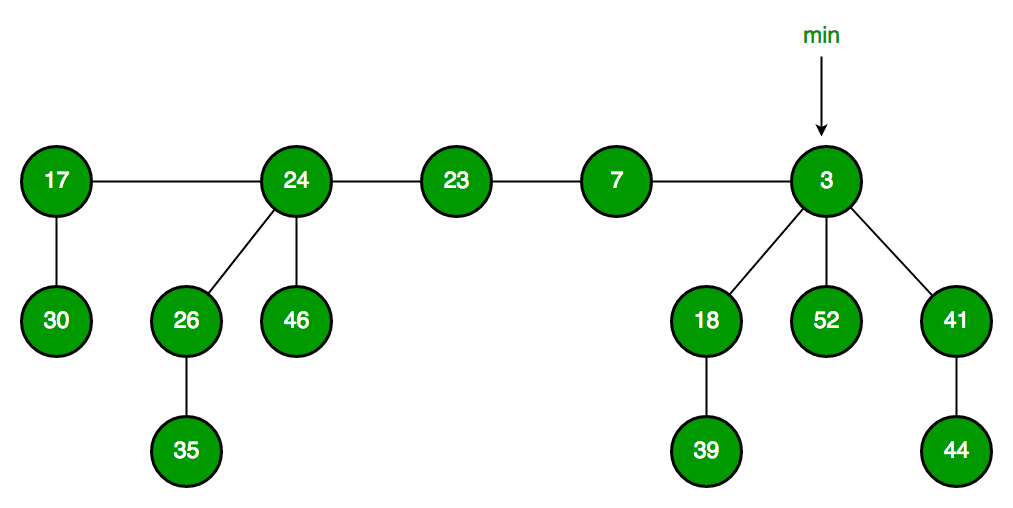Related Articles

# Fibonacci Heap | Set 1 (Introduction)

• Difficulty Level : Medium
• Last Updated : 16 Jul, 2021

Heaps are mainly used for implementing priority queue. We have discussed below heaps in previous posts.

In terms of Time Complexity, Fibonacci Heap beats both Binary and Binomial Heaps.

Below are amortized time complexities of Fibonacci Heap

```1) Find Min:      Θ(1)     [Same as both Binary and Binomial]
2) Delete Min:    O(Log n) [Θ(Log n) in both Binary and Binomial]
3) Insert:        Θ(1)     [Θ(Log n) in Binary and Θ(1) in Binomial]
4) Decrease-Key:  Θ(1)     [Θ(Log n) in both Binary and Binomial]
5) Merge:         Θ(1)     [Θ(m Log n) or Θ(m+n) in Binary and
Θ(Log n) in Binomial]```

Like Binomial Heap, Fibonacci Heap is a collection of trees with min-heap or max-heap property. In Fibonacci Heap, trees can have any shape even all trees can be single nodes (This is unlike Binomial Heap where every tree has to be Binomial Tree).

Below is an example Fibonacci Heap taken from hereFibonacci Heap maintains a pointer to minimum value (which is root of a tree). All tree roots are connected using circular doubly linked list, so all of them can be accessed using single ‘min’ pointer.

The main idea is to execute operations in “lazy” way. For example merge operation simply links two heaps, insert operation simply adds a new tree with single node. The operation extract minimum is the most complicated operation. It does delayed work of consolidating trees. This makes delete also complicated as delete first decreases key to minus infinite, then calls extract minimum.

Below are some interesting facts about Fibonacci Heap

1. The reduced time complexity of Decrease-Key has importance in Dijkstra and Prim algorithms. With Binary Heap, time complexity of these algorithms is O(VLogV + ELogV). If Fibonacci Heap is used, then time complexity is improved to O(VLogV + E)
2. Although Fibonacci Heap looks promising time complexity wise, it has been found slow in practice as hidden constants are high (Source Wiki).
3. Fibonacci heap are mainly called so because Fibonacci numbers are used in the running time analysis. Also, every node in Fibonacci Heap has degree at most O(log n) and the size of a subtree rooted in a node of degree k is at least Fk+2, where Fk is the kth Fibonacci number.

We will soon be discussing Fibonacci Heap operations in detail.